Next: Spherical Pendulum Up: Lagrangian Dynamics Previous: Sliding down a Sliding

Generalized Momenta

Consider the motion of a single particle moving in one dimension. The kinetic energy is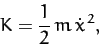(649)

where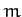is the mass of the particle, andits displacement. Now, the particle's linear momentum is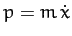. However, this can also be written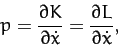(650)

since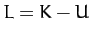, and the potential energy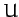is independent of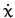.

Consider a dynamical system described by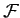generalized coordinates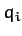, for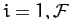. By analogy with the above expression, we can define generalized momenta of the form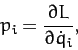(651)

for. Here,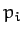is sometimes called the momentum conjugate to the coordinate. Hence, Lagrange's equation (613) can be written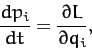(652)

for. Note that a generalized momentum does not necessarily have the dimensions of linear momentum.

Suppose that the Lagrangian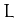does not depend explicitly on some coordinate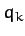. It follows from Equation (652) that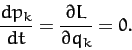(653)

Hence,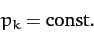(654)

The coordinateis said to be ignorable in this case. Thus, we conclude that the generalized momentum associated with an ignorable coordinate is a constant of the motion.

For example, in Section 9.5, the Lagrangian (615) for a particle moving in a central potential is independent of the angular coordinate. Thus,is an ignorable coordinate, and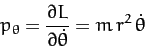(655)

is a constant of the motion. Of course,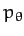is the angular momentum about the origin. This is conserved because a central force exerts no torque about the origin.

Again, in Section 9.7, the Lagrangian (642) for a mass sliding down a sliding slope is independent of the Cartesian coordinate. It follows thatis an ignorable coordinate, and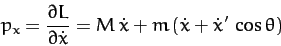(656)

is a constant of the motion. Of course,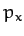is the total linear momentum in the-direction. This is conserved because there is no external force acting on the system in the-direction.Next: Spherical Pendulum Up: Lagrangian Dynamics Previous: Sliding down a Sliding
Richard Fitzpatrick 2011-03-31